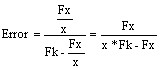# Making Accurate Frequency Measurements

## Overview

How accurate are the frequency measurements you make using National Instruments counters? This document describes three methods for making frequency measurements with NI 660x counter/timer devices and explains how to determine the accuracy of your frequency measurements.

## Sources of Frequency Measurement Error

There are two sources of error in frequency measurements:

• Errors in the frequency of the crystal oscillator
• Measurement error

The total measurement error is the sum of these two sources, or:

Measurement Error = Crystal Oscillator Error + Measurement Error

## Crystal Oscillator Error

The crystal oscillator we use on the PCI-6601 and PCI-6602 is rated at 75 parts per million (ppm) stability over temperature. The crystal oscillator we use for the PXI-6602 is 200 ppm, but when in the PXI chassis, PXI 6602 will automatically phase lock to the clock of PXI backplane, which as accuracy of 75 ppm. With phase locking enabled the PXI 6602 will have 75 ppm accuracy as well.

75 ppm means that for every 1 million Hertz, the frequency can be off by +/- 75 Hz. For a 6601 board, which has a 20 MHz oscillator, the error due to crystal used could be up to +/- 75Hz/1MHz*20= +/-1500 Hz. For the 6602 board, which has an 80 MHz oscillator, the error is +/- 6000 Hz.

The 10 MHz Oven Controlled Crystal Oscillator (OCXO) on a 6608 board has a frequency stability of 75 ppb over one year. This means that the frequency can be off by 0.75 Hz in 10 MHz over a period of one year. When the 6608 board is in slot 2 of the PXI chassis, NI-DAQ software automatically drives the 10 MHz clock from the OCXO to replace the PXI backplane clock. This allows other devices with phase clock loop circuitry to take advantage of the accuracy of the 6608 OCXO. For an example, if a PXI 6602 is in the same PXI chassis, its 80 MHz timebase now has the error of +/- 6Hz, comparing to +/- 6000 Hz. One important note here is that in order for the PXI-6608 to drive and replace the PXI backplane clock, it must be in slot 2, the slot next to the controller, of the PXI chassis. If the PXI-6608 is in any other slots, then the crystal error will be the same as PXI-6602.

For more information about how the PXI-6608 clock is shared, please refer to the Note to User: About Your 6608 Device. It describes the OCXO, and has a diagram that helps demonstrate how the PXI-6608 improves clock stability of its own board clock and that of other modules.

## Measurement Error and Three Frequency Measurement Methods

Measurement errors are inherent in frequency measurements, but you can minimize the effects of measurement error by choosing a frequency measurement method that is most suited for the frequencies you are measuring and the timebase of your NI 660x device. In this section we describe three ways to make frequency measurements and explain the effects that measurement error can have on the accuracy of measurements made with each method.

The three frequency measurement methods described below are:
Method 1 -- Inverse Period Measurement
Method 2 -- Count Number of Pulses in Known Time
Method 3 -- Measure Time of Known Number of Cycles

The three example VIs attached to this document demonstrate these three methods as follows:
Frequency 123.vi lets you use any of the three methods.
Frequency Method 2.vi counts pulses for a specified amount of time (Method 2).
Frequency Method 3.vi measures the time for a specified number of cycles (Method 3).

METHOD 1: Inverse Period Measurement
Example: The period measurement example that comes with LabVIEW, or Frequency 123.vi at Making Frequency Measurements with NI 660x Devices.

The simplest method is period measurement. You can measure the period by counting the rising or falling edges of a known source frequency between the two consecutive rising or falling edges of the unknown frequency. By taking the frequency of the known source and dividing by the count, you can calculate the period of the unknown signal. Typically one of the internal timebase, 20 MHz or 80 MHz, is used, but external clock source can also be used as long as it is less than or equal to the maximum source frequency supported by the hardware.

This type of measurement is suitable for low frequency measurement. Suppose your signal to be measured is a perfect 50 kHz frequency and you are measuring it using a 20 MHz internal timebase. Assuming that the 20 MHz timebase is perfect, in other words the crystal oscillator used to generate the 20 MHz timebase is perfect, you should count 400 edges between two consecutive rising or falling edges of the perfect 50 kHz signal. However, due to the fact that we cannot control the phase relationship between the two signals, the count can be off by +/- 1. This is called the measurement error. Thus, the counter will return 399, 400, or 401. Doing Math gives the following results:

 Count returned: 399 400 401 Calculated frequency (kHz): 50.13 50 49.88

The deviation from the actual frequency (50 kHz) resulting from the measurement error is not too significant. In fact, if an 80 MHz timebase is used, that deviation will be even smaller.

 Count returned: 1599 1600 1601 Calculated frequency (kHz): 50.03 50 49.97

If the frequency to be measured was 5 MHz instead, using the same 20 MHz source frequency, then the counter should return a count of 4. Because of the measurement error, the count could be +/- 1, so you can also expect a count of 3 or 5. Doing math gives the following results:

 Count returned: 3 4 5 Calculated frequency (MHz): 6.67 5 4

You can see that a difference of one count results in a larger sampling error as the frequency to be measured increases. Even with an 80 MHz timebase, we would still have significant deviation from the actual frequency:

 Count returned: 15 16 17 Calculated frequency (MHz): 5.33 5 4.7

To calculate measurement error using this frequency measurement method, we have the following formula: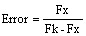See the calculations below for more details.

METHOD 2: Count Number of Pulses in Known Time
Example: Frequency Method 2.vi or Frequency 123.vi at Making Frequency Measurements with NI 660x Devices.

Method 1 works well as long as the frequency of the signal to be measured is significantly slower than the known source frequency. As the frequency of the signal to be measured increases to approach the frequency of the source frequency, measurement error increases. To improve the accuracy of the frequency measurement for higher unknown frequency, we can use this second measurement method, count number of pulses in known time. In this configuration, the counter will count number of unknown high frequency during a period of known signal. The frequency can be calculated by multiplying the count by the frequency of the unknown signal.

To use the previous example, frequency to be measured is 5 MHz. The counter will count rising edges of the 5 MHz during a period of a known frequency. Assuming that we select that frequency to be 10 Hz and it is generated from a perfect timebase (without crystal oscillator error). This means that during the two consecutive edges of the known frequency, which is 0.1 second, we should count 500,000 edges. Because we still cannot control the phase relationship between our 10 Hz and 5 MHz signals, we will still have measurement error of +/- 1 count. Doing the math will give us the following results:

 Count returned: 499999 500000 500001 Calculated frequency (MHz): 4.99999 5 5.00001

Compare this measurement result to the result calculated by the previous measurement method, we can see that the deviation is much reduced. The deviation can be further improved by using a lower frequency known signal. Let's redo the calculation using 1 Hz known frequency:

 Count returned: 499999 500000 500001 Calculated frequency (MHz): 4.99999 5 5.00001

To summarize, by using two counters, you can get more accurate frequency measurement for higher frequencies. The larger the period of the known signal used to gate the counting, the smaller the measurement error. The measurement error for this measurement method: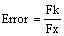See the calculations below for more details.

METHOD 3: Measure Time of Known Number of Cycles
Example: Frequency Method 3.vi or Frequency 123.vi at Making Frequency Measurements with NI 660x Devices.

So far, we've discussed two measurement methods, inverse period and count number of pulses in known time. The inverse period measurement method is suitable for lower frequency measurements while the second measurement method can achieve higher accuracy with higher frequency signals. However, if your application involves measuring a pulse width modulated signal and the span of the frequency range covers both low and high frequencies, then this third measurement method can be considered.

Similar to measurement method 2, count number of pulses in known time, this measurement method also uses two counters. The first counter is used to divide down the frequency of the signal to be measured, then the second counter is used to measure the period of the divide down frequency. The actual frequency can be calculated by multiplying the resulting frequency measurement by the divide down value. With this frequency method, the larger the divide down value, the slower the resulting frequency, and more accurate the measurement result.

The calculation of measurement error for this measurement method is similar to method 1: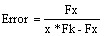See the calculations below for more details.

## Which Method is Best?

The best method will depend on the type of frequency your wish to measure. Period measurement is the simplest method and it requires only one counter; however, as frequencies increase, the measurement error increases. The second and third measurement methods both use 2 counters. The second method (gate a counter for a known amount of time and count the number of edges) is more generic and is not suitable for lower frequencies unless you use very long gate times to measure these lower frequencies.

The third method offers better accuracy for the same measurement time, but is not as generic, since you need to specify how many cycles to make a measurement over. It is important to know what frequency you expect to measure with this method. For instance, for a 10 MHz signal, you will use 10 million cycles for a 1 second measurement time. For a 1 MHz signal, using 10 million cycles will require 10 seconds for a measurement. In order to complete this measurement on a 1 MHz signal in one second, you will want to make the measurement over 1 million cycles.

## Calculations

Fk = known source frequency
Fx = frequency to be measured if no error
Fm= frequency measured

Measurement Error Calculation for Measurement Method 1:
Fk >> Fx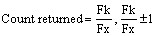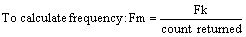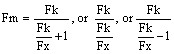After simplification: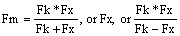To calculate measurement error: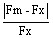If Fm=Fx: Error=0
If Fm>Fx: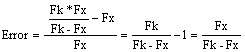If Fm<Fx: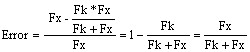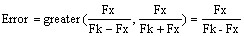Measurement Error Calculation for Measurement Method 2:

Fk << Fx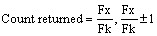To calculate frequency: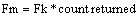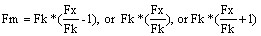After simplification:
Fm=Fx - Fk, or FK, or Fx, or Fx + Fk

To calculate measurement error: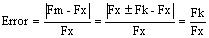Measurement Error Calculation for Measurement Method 3:

Fk >> Fx/x

Calculation for measurement error is identical to method 1. The only difference is that we now need to divide frequency to be measured, Fx, by an integer value x: